Data Structures and Algorithms with Object-Oriented Design Patterns in C## Exercises

1.   The array-based stack implementation introduced in Programuses a fixed length array. As a result, it is possible for the stack to become full.
1. Rewrite the Push method so that it doubles the length of the array when the array is full.
2. Rewrite the Pop method so that it halves the length of the array when the array is less than half full.
3. Show that the average time for both push and pop operations is O(1). Hint: Consider the running time required to push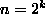items onto an empty stack, where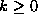.
2. Consider a sequence S of push and pop operations performed on a stack that is initially empty. The sequence S is a valid sequence of operations if at no point is a pop operation attempted on an empty stack and if the stack is empty at the end of the sequence. Design a set of rules for generating a valid sequence.
3. Devise an implementation of the queue abstract data type using two stacks. Give algorithms for the Enqueue and Dequeue operations, and derive tight big-oh expressions for the running times of your implementation.
4. Write each of the following infix expressions in postfix notation:
1.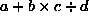,
2.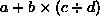,
3.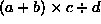,
4.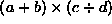,
5.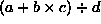, and
6.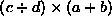.
5. Write each of the following postfix expressions in infix notation:
1.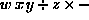,
2.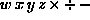,
3.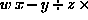,
4.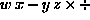,
5., and
6.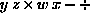.
6.   Devise an algorithm which translates a postfix expression to a prefix expression. Hint: Use a stack of strings.
7. The array-based queue implementation introduced in Programuses a fixed length array. As a result, it is possible for the queue to become full.
1. Rewrite the Enqueue method so that it doubles the length of the array when the array is full.
2. Rewrite the Dequeue method so that it halves the length of the array when the array is less than half full.
3. Show that the average time for both enqueue and dequeue operations is O(1).
8. Stacks and queues can be viewed as special cases of deques. Show how all the operations on stacks and queues can be mapped to operations on a deque. Discuss the merits of using a deque to implement a stack or a queue.
9. Suppose we add a new operation to the stack ADT called FindMinimum that returns a reference to the smallest element in the stack. Show that it is possible to provide an implementation for FindMinimum that has a worst case running time of O(1).
10.   The breadth-first traversal method shown in Programvisits the nodes of a tree in the order of their levels in the tree. Modify the algorithm so that the nodes are visited in reverse. Hint: Use a stack.Copyright © 2001 by Bruno R. Preiss, P.Eng. All rights reserved.